# Form Tool Calculation ProgramForm Tool Design Manual

## FEATURES

• Eliminates long, complicated manual calculations.
• Eliminates corrected diameter tables, charts, graphs, trig tables, and square root calculations.
• Calculates any Circular Form Tool - regardless of Diameter, Offset or Top Rake.
• Calculates any Flat Type Form Tool - regardless of Front Clearance Angle or Top Rake.
• Can print out answers for a permanent record.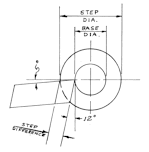#### FOR RESHARPENABLE QUICK CHANGE FORM TOOL INSERTS

All you need to do is enter:
• Front Clearance Angle = 12º
• Top Rake Angle = 5º
• Base Diameter of Part =

Then as you enter each part diameter, the program calculates the corrected step difference.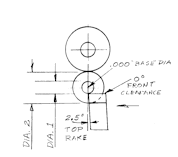#### FOR RESHARPENABLE QUICK CHANGE SHAVE TOOL INSERTS

All you need to do is enter:
• Front Clearance Angle = 0º
• Top Rake Angle = 0º
• Base Diameter of Part = .000",.125" or .500" depending on holder being used

Then as you enter each part diameter, the program calculates the corrected step difference.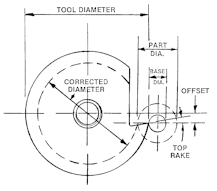#### FOR CIRCULAR FORM TOOLS

All you need to do is enter:
• Tool Diameter ---
• Tool Offset Distance---
• Top Rake Angle---
• Base Diameter of Part---
Then as you enter each part diameter, the program calculates tool diameter and step difference.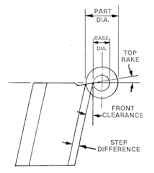#### FOR FLAT TYPE FORM TOOLS

All you need to do is enter:
• Front Clearance Angle ---
• Top Rake Angle---
• Base Diameter of Part---
Then as you enter each part diameter, the program calculates the corrected step difference.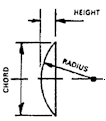All you need to do is enter:
• Enter any two of the three dimensions of a chord.
The program will calculate the third dimension.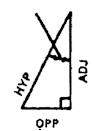#### FOR TRIANGLE PROBLEMS

All you need to do is enter:
• Any two of the four dimensions of a right angle triangle.
The program will calculate the remaining two dimensions.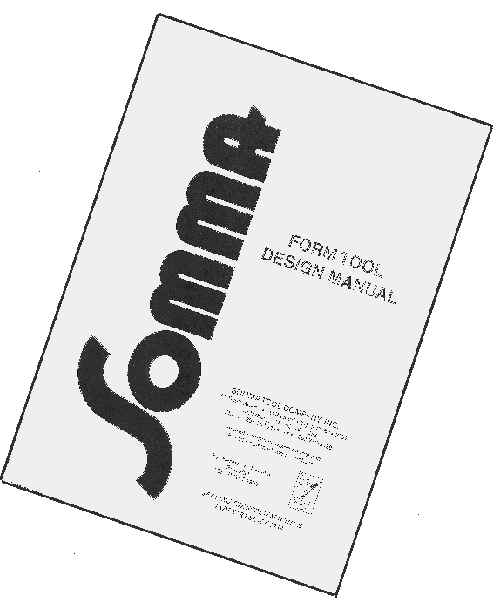• CHECKLIST
• DRAWING
• CALCULATING
• DIMENSIONING